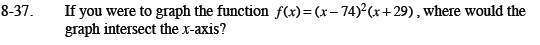### Home > CCA2 > Chapter Ch8 > Lesson 8.1.2 > Problem8-37

8-37.

If you were to graph the function f(x) = (x − 74)2(x + 29), where would the graph intersect the x-axis? Homework Help ✎Remember that wherever the graph intersects the x-axis, f(x) will be zero. Use the Zero Product Property.

The graph has a double root at (74, 0) and another root at (-29, 0).